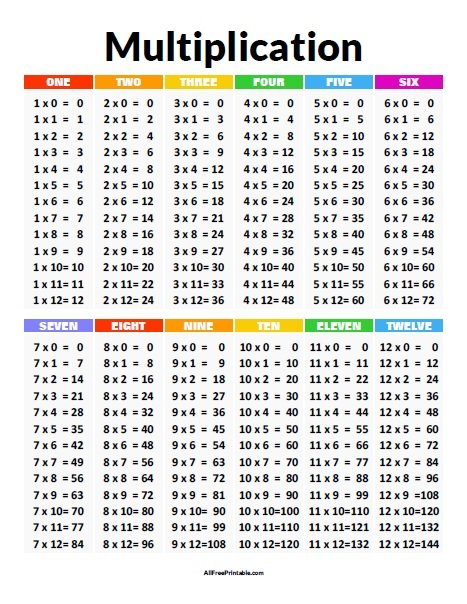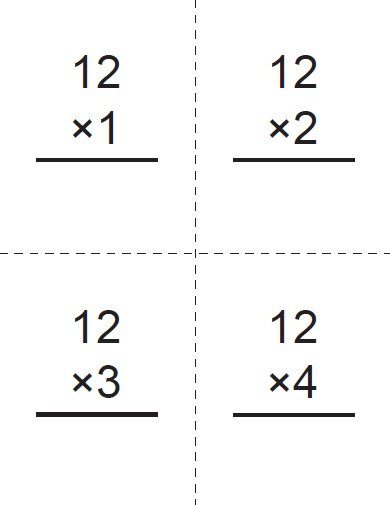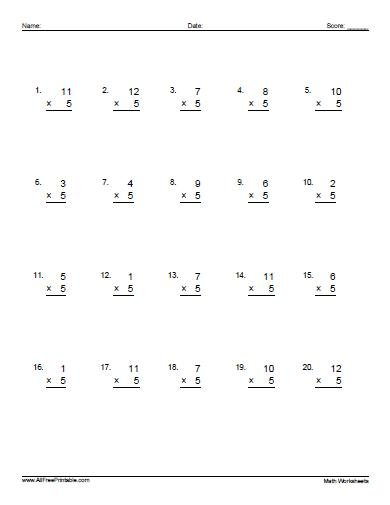# Multiplication## Multiplication Chart

By
|
In Math Worksheets , Multiplication , School
|
On August 31, 2021
Free Printable Multiplication Chart. Free Printable Multiplication Chart, a great resource to help kids learn and remember the multiplication tables at early grades at school or homeschool. Teacher, Parents, and Tutors can use the multiplication table to hand out to students for easy multiplication table...

## Multiplication Times Table Chart

By
|
On May 26, 2015

Free Printable Multiplication Times Table Chart. Free Printable Multiplication Times Table Chart, a great free resource to help kids learn and practice the multiplication tables at summer school. You can.

## Multiplication Facts

By
|
On May 26, 2015

Free Printable Multiplication Facts. Free Printable Multiplication Facts, a great reference sheet to help kids learn and practice the multiplication tables this summer vacations. Teachers, Parents, and Tutors can use.## Multiplication Flash Cards

By
|
In Multiplication
|
On January 29, 2014
Free Printable Multiplication Flash Cards. Free Printable Multiplication Flash Cards of time tables 1 – 12 with answers. Great for teachers at school or parents at home that wants to help kids improve multiplication skills. Print the Multiplication Flash Cards you need free using a...

## Basic Multiplication Problems

By
|
On September 24, 2013

Free Printable Basic Multiplication Problems. Free Printable Basic Multiplication Problems, a great free printable for primary school. Print the Basic Multiplication Problems free using your home laser or inkjet printer,.

## Multiplication Problems

By
|
On May 29, 2013

Free Printable Multiplication Problems. Free Printable Multiplication Problems, for starter students to advanced. Can be of great help when teaching multiplication at school or home. Print the Multiplication Problems free.## Multiplication Worksheets

By
|
In Math Worksheets , Multiplication , School
|
On May 29, 2013
Free Printable Multiplication Worksheets. Free Printable Multiplication Worksheets, from basic multiplication tables and digits to more advanced problems. Great for teachers at school or parents at home. Print the Multiplication Worksheets you need free using a laser or inkjet printer, and give kids for math...

## Multiplying by 10 Worksheets

By
|
On May 27, 2013

Free Printable Multiplying by 10 Worksheets. Free Printable Multiplying by 10 Worksheets to improve your kids math skills. Use for a practice, quiz or for a multiplying by 10 math.

## Multiplying by 9 Worksheets

By
|
On May 27, 2013

Free Printable Multiplying by 9 Worksheets. Printable Multiplying by 9 Worksheets you can use to improve your kids math skills at home. Use for a quiz, test or just to.

This div height required for enabling the sticky sidebar